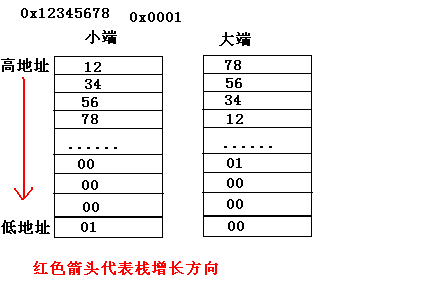# 栈增长方向与大端/小端问题

#include<stdio.h>
static int stack_dir;
static void find_stack_direction (void)  {
static char   *addr = NULL;   /* address of first
`dummy', once known
*/
char     dummy;          /* to get stack address */

if (addr == NULL)
{                           /* initial entry */
addr = &dummy;

find_stack_direction ();  /* recurse once */
}
else                          /* second entry */
if (&dummy > addr)
stack_dir = 1;            /* stack grew upward */
else
stack_dir = -1;           /* stack grew downward */
}

int main(void)
{
find_stack_direction();
if(stack_dir==1)
puts("stack grew upward");
else
puts("stack grew downward");
return 0;

find_stack_direction函数使用函数递归的方法

int x=1;
if(*(char*)&x==1)
printf("little-endian\n");
else
printf("big-endian\n");

union{
int i;
char c;
}x;
x.i=1;
if(x.c==1)
printf("little-endian\n");
else
printf("big-endian\n");int chgendian(int x){
int x2=0;
char *p=(char*)&x;
int i=sizeof(int)-1;
while(i>=0){
x2|=*p<<(i*8);
i--;
p++;
}
return x2;
}

posted @ 2012-06-22 20:47  Mr.Rico  阅读(13235)  评论(2编辑  收藏  举报